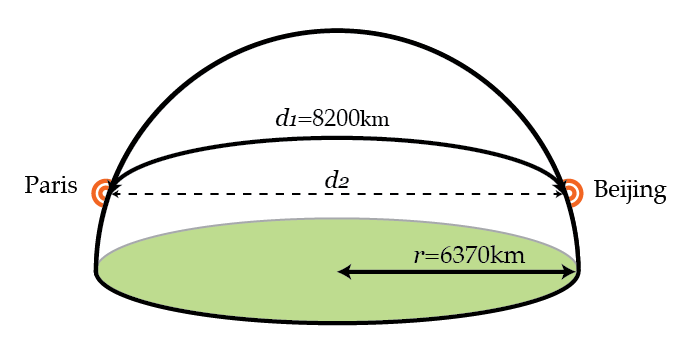# Headed to Beijing, back for dinner!The gravity train is a hypothetical idea proposed by Robert Hooke (he of Hooke's law fame) to Isaac Newton in the 1600's. It consists of a simple idea that's hard to implement in practice. Dig a tunnel that runs straight through Earth between two points on the surface. If you can figure out how to remove friction and lower air resistance, you now have a mechanism for extremely efficient and rapid travel between widely separated points. Simply drop something into the tunnel at one end. Gravity will initially pull it downwards through the tunnel, eventually reaching high speeds. Once the object is halfway through the tunnel, gravity will now slow it back down, so you can retrieve the object easily on the other side.

While there are obvious engineering impracticalities, it winds up being pretty amazing how fast a gravity train can get things from point to point without needing fuel. So, let's say we wanted to go from Beijing to Paris using such a gravity train. How long does the trip take assuming a frictionless and drag-free train to the nearest minute?

Earth can be modeled as a sphere of uniform density, with total mass $6 \times 10^{24}~\mbox{kg}$ and radius $6370~\mbox{km}$. The shortest distance from Beijing to Paris on the surface of Earth is approximately $8200~\mbox{km}$.

Details and Assumptions:

• Newton's constant is $6.67 \times 10^{-11}~\mbox{N m}^2/\mbox{kg}^2$.
• The mass of the Earth is $6 \times 10^{24}~\mbox{kg}$.
×